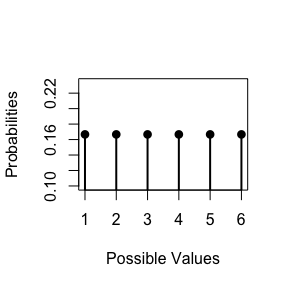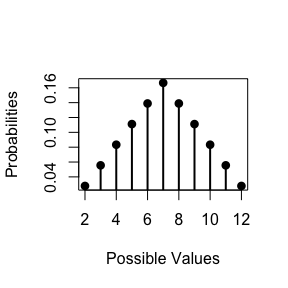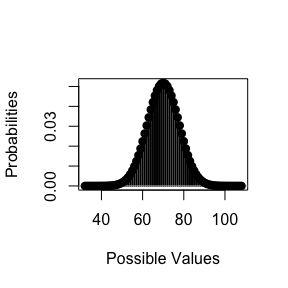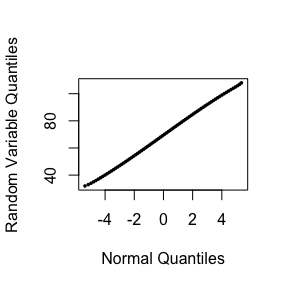discreteRV is an R package that allows simple creation and manipluation of discrete random variables using a clean and familiar syntactical interface. This vignette will guide you through the basic operations supported by discreteRV.

## Creating discrete random variables

A discrete random variable is defined through the use of the function. accepts a vector of outcomes, a vector of probabilities, and returns an object. The code to create X, a random variable representing the roll of a fair die, is as follows:

(X <- RV(outcomes = 1:6, probs = 1/6))
## Random variable with 6 outcomes
##
## Outcomes   1   2   3   4   5   6
## Probs    1/6 1/6 1/6 1/6 1/6 1/6

Probabilities are uniform by default, so the following code is equivalent:

(X <- RV(1:6))
## Random variable with 6 outcomes
##
## Outcomes   1   2   3   4   5   6
## Probs    1/6 1/6 1/6 1/6 1/6 1/6

Outcomes can be specified as a range of values, which is useful for distributions in which the outcomes that can occur with non-zero probability are unbounded. To define a Poisson random variable Y, we specify the outcomes as a range and the probabilities as a function:

pois.func <- function(y, lambda) { return(lambda^y * exp(-lambda) / factorial(y)) }

(Y <- RV(outcomes = c(0, Inf), probs = pois.func, lambda = 2))
## Random variable with outcomes from 0 to Inf
##
## Outcomes     0     1     2     3     4     5     6     7     8     9    10    11
## Probs    0.135 0.271 0.271 0.180 0.090 0.036 0.012 0.003 0.001 0.000 0.000 0.000
##
## Displaying first 12 outcomes

Several common distributions are natively supported so that the function need not be defined manually. For instance, an equivalent method of defining Y is:

(Y <- RV("poisson", lambda = 2))
## Random variable with outcomes from 0 to Inf
##
## Outcomes     0     1     2     3     4     5     6     7     8     9    10    11
## Probs    0.135 0.271 0.271 0.180 0.090 0.036 0.012 0.003 0.001 0.000 0.000 0.000
##
## Displaying first 12 outcomes

The RV function also allows the definition of a random variable in terms of odds. We construct a loaded die in which a roll of one is four times as likely as any other roll as:

(X.loaded <- RV(outcomes = 1:6, odds = c(4, 1, 1, 1, 1, 1)))
## Random variable with 6 outcomes
##
## Outcomes   1   2   3   4   5   6
## Odds     4:5 1:8 1:8 1:8 1:8 1:8

## Probability Calculations

Basic probability calculations are performed using the $$P$$ function, such as:

P(X == 2)
##  0.1666667
P(X < 3)
##  0.3333333
P(X < 3 | X < 4)
##  0.6666667

Consider our Poisson random variable Y, and suppose we want to obtain the probability that Y is within a distance $$\delta$$ of its mean parameter $$\lambda = 2$$:

delta <- 3
lambda <- 2

P((Y >= lambda - delta) %AND% (Y <= lambda + delta))
##  0.9834364

Alternatively, we could have also used the slightly more complicated looking expression:

P((Y - lambda)^2 <= delta^2)
##  0.9834364

We can compute several other distributional quantities, including the expected value and the variance of a random variable:

E(X)
##  3.5
V(X)
##  2.916667
E( (X-E(X))^2 )
##  2.916667

## Joint Distributions

A joint distribution can be defined with the jointRV function, with a list of possible outcomes and probabilities in row-major order:

(AandB <- jointRV(outcomes = list(1:3, 0:2), probs = 1:9 / sum(1:9)))
## Random variable with 9 outcomes
##
## Outcomes  1,0  1,1  1,2  2,0  2,1  2,2  3,0  3,1  3,2
## Probs    1/45 2/45 1/15 4/45  1/9 2/15 7/45 8/45  1/5

The individual marginal distributions can be obtained by use of the marginal function:

A <- marginal(AandB, 1)
B <- marginal(AandB, 2)

Although the marginal distributions allow all the same computations of any univariate random variable, they maintain a special property. The joint distribution that produced the marginals is stored as attributes in the object. This allows for several more advanced probability calculations, involving the marginal and conditional distributions:

P(A < B)
##  0.06666667
P(A == 2 | B > 0)
##  0.3333333
P(A == 2 | (B == 1) %OR% (B == 2))
##  0.3333333
independent(A, B)
##  FALSE
A | (A > 1)
## Random variable with 2 outcomes
##
## Outcomes    2    3
## Probs    5/13 8/13
A | (B == 2)
## Random variable with 3 outcomes
##
## Outcomes   1   2   3
## Probs    1/6 1/3 1/2
E(A | (B == 2))
##  2.333333

A joint distribution can be defined from a univariate distribution using the iid function (to create a joint distribution of n independent realizations of the specified random variable) of the SofIID function (to create a distribution for the sum of n independent realizations of the random variable):

(X2 <- iid(X, n = 2))
## Random variable with 36 outcomes
##
## Outcomes  1,1  1,2  1,3  1,4  1,5  1,6  2,1  2,2  2,3  2,4  2,5  2,6
## Probs    1/36 1/36 1/36 1/36 1/36 1/36 1/36 1/36 1/36 1/36 1/36 1/36
##
## Displaying first 12 outcomes
(X3 <- iid(X, n = 3))
## Random variable with 216 outcomes
##
## Outcomes 1,1,1 1,1,2 1,1,3 1,1,4 1,1,5 1,1,6 1,2,1 1,2,2 1,2,3 1,2,4 1,2,5 1,2,6
## Probs    1/216 1/216 1/216 1/216 1/216 1/216 1/216 1/216 1/216 1/216 1/216 1/216
##
## Displaying first 12 outcomes
(X2 <- SofIID(X, n = 2))
## Random variable with 11 outcomes
##
## Outcomes    2    3    4    5    6    7    8    9   10   11   12
## Probs    1/36 1/18 1/12  1/9 5/36  1/6 5/36  1/9 1/12 1/18 1/36
(X20 <- SofIID(X, n = 20, progress = FALSE))
## Random variable with 77 outcomes
##
## Outcomes 32 33 34 35 36 37 38 39 40 41 42 43
## Probs     0  0  0  0  0  0  0  0  0  0  0  0
##
## Displaying first 12 outcomes

Alternatively, the + and * operators have been overloaded to allow these computations in a cleaner syntax, such as:

RV(1:6) + RV(1:6)
## Random variable with 11 outcomes
##
## Outcomes    2    3    4    5    6    7    8    9   10   11   12
## Probs    1/36 1/18 1/12  1/9 5/36  1/6 5/36  1/9 1/12 1/18 1/36

## Plotting

Plot and qqnorm methods are defined for random variable objects:

plot(X)plot(X2)plot(X20)qqnorm(X20)
abline()## Simulation

discreteRV also includes a set of functions to simulate trials from a random variable.

(X.sim <- rsim(X, 10))
## Simulated Vector:  3 3 5 6 3 4 1 2 3 6
##
## Random variable with 6 outcomes
##
## Outcomes   1   2   3   4   5   6
## Probs    1/6 1/6 1/6 1/6 1/6 1/6
props(X.sim)
## RV
##   1   2   3   4   5   6
## 0.1 0.1 0.4 0.1 0.1 0.2
Prop(X.sim == 3)
##  0.4
Prop(X.sim > 3)
##  0.4

Craps is a common dice game played in casinos. The game begins with what is called the “Come Out” roll, in which two fair dice are rolled. If a sum of seven or eleven is obtained, the player wins. If a sum of two, three, or twelve is obtained, the player loses. In all other cases, the roll obtained is declared the Point" and the player rolls again in an attempt to obtain this same point value. If the player rolls the Point, they win, but if they roll a seven, they lose. Rolls continue until one of these two outcomes is achieved.

discreteRV allows for a seamless analysis and simulation of the probabilities associated with different events in Craps. Let us begin by asking “What is the probability that the game ends after the first roll?” To answer this question we construct two random variables. We note that calling returns a random variable for a single roll of a fair die, and then we use the overloaded operator to sum over two rolls to obtain the random variable .

(Roll <- RV(1:6) + RV(1:6))
## Random variable with 11 outcomes
##
## Outcomes    2    3    4    5    6    7    8    9   10   11   12
## Probs    1/36 1/18 1/12  1/9 5/36  1/6 5/36  1/9 1/12 1/18 1/36

Recall that the game ends after the first roll if and only if a seven or eleven is obtained (resulting in a win), or a two, three, or twelve is obtained (resulting in a loss). Hence, we calculate the probability that the game ends after the first roll as follows:

P(Roll %in% c(7, 11, 2, 3, 12))
##  0.3333333

Now suppose we would like to condition on the game having ended after the first roll. Using the conditional probability operator in discreteRV, we can obtain the probabilities of winning and losing given that the game ended after the first roll:

P(Roll %in% c(7, 11) | Roll %in% c(7, 11, 2, 3, 12))
##  0.6666667
P(Roll %in% c(2, 3, 12) | Roll %in% c(7, 11, 2, 3, 12))
##  0.3333333

Now, let’s turn our attention to calculating the probability of winning a game in two rolls. Recall that we can use the function to generate joint distributions of independent and identically distributed random variables. In this case, we would like to generate the joint distribution for two independent rolls of two dice. Now, we will have $$11^2$$ possible outcomes, and our job is to determine which outcomes result in a win. We know that any time the first roll is a seven or eleven, we will have won. We also know that if the roll is between four and ten inclusive, then we will get to roll again. To win within two rolls given that we’ve received a four through ten requires that the second roll match the first. We can enumerate the various possibilities to calculate the probability of winning in two rolls, which is approximately 30%.

TwoRolls <- iid(Roll, 2)

First <- marginal(TwoRolls, 1)
Second <- marginal(TwoRolls, 2)

P(First %in% c(7, 11) %OR% (First %in% 4:10 %AND% (First == Second)))
##  0.2993827

Finally, suppose we are interested in the empirical probability of winning a game of Craps. Using the simulation functions in discreteRV, we can write a routine to simulate playing Craps. Using the function, we simulate a single game of Craps by rolling from our random variable , which represents the sum of two dice. We then perform this simulation 100000 times. The results indicate that the player wins a game of craps approximately 49% of the time.

craps_game <- function(RV) {

my.roll <- rsim(RV, 1)

if (my.roll %in% c(7, 11)) { return(1) }
else if (my.roll %in% c(2, 3, 12)) { return(0) }
else {
new.roll <- 0
while (new.roll != my.roll & new.roll != 7) {
new.roll <- rsim(RV, 1)
}

return(as.numeric(new.roll == my.roll))
}
}

sim.results <- replicate(100000, craps_game(Roll))
mean(sim.results)
##  0.49325